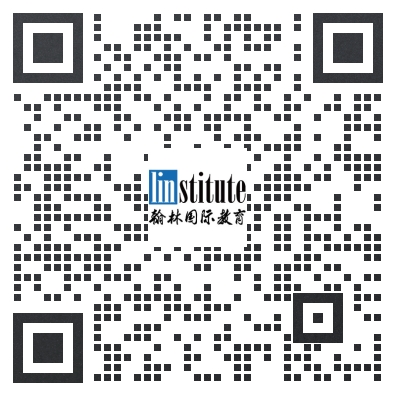# 2013 USAMO Problems真题及答案

+答案解析请参考文末

## Day 1

### Problem 1

In triangle$ABC$, points$P,Q,R$ lie on sides$BC,CA,AB$ respectively. Let$\omega_A$$\omega_B$$\omega_C$ denote the circumcircles of triangles$AQR$$BRP$$CPQ$, respectively. Given the fact that segment$AP$ intersects$\omega_A$$\omega_B$$\omega_C$ again at$X,Y,Z$ respectively, prove that$YX/XZ=BP/PC$.

### Problem 2

For a positive integer$n\geq 3$ plot$n$ equally spaced points around a circle. Label one of them$A$, and place a marker at$A$. One may move the marker forward in a clockwise direction to either the next point or the point after that. Hence there are a total of$2n$ distinct moves available; two from each point. Let$a_n$ count the number of ways to advance around the circle exactly twice, beginning and ending at$A$, without repeating a move. Prove that$a_{n-1}+a_n=2^n$ for all$n\geq 4$.

### Problem 3

Let$n$ be a positive integer. There are$\tfrac{n(n+1)}{2}$ marks, each with a black side and a white side, arranged into an equilateral triangle, with the biggest row containing$n$ marks. Initially, each mark has the black side up. An operation is to choose a line parallel to the sides of the triangle, and flipping all the marks on that line. A configuration is called admissible if it can be obtained from the initial configuration by performing a finite number of operations. For each admissible configuration$C$, let$f(C)$ denote the smallest number of operations required to obtain$C$ from the initial configuration. Find the maximum value of$f(C)$, where$C$ varies over all admissible configurations.

## Day 2

### Problem 4

Find all real numbers$x,y,z\geq 1$ satisfying$$\min(\sqrt{x+xyz},\sqrt{y+xyz},\sqrt{z+xyz})=\sqrt{x-1}+\sqrt{y-1}+\sqrt{z-1}.$$

### Problem 5

Given positive integers$m$ and$n$, prove that there is a positive integer$c$ such that the numbers$cm$ and$cn$ have the same number of occurrences of each non-zero digit when written in base ten.

### Problem 6

Let$ABC$ be a triangle. Find all points$P$ on segment$BC$ satisfying the following property: If$X$ and$Y$ are the intersections of line$PA$ with the common external tangent lines of the circumcircles of triangles$PAB$ and$PAC$, then$$\left(\frac{PA}{XY}\right)^2+\frac{PB\cdot PC}{AB\cdot AC}=1.$$

### 2013USAMO真题参考答案及详解## 报名及辅导请联系